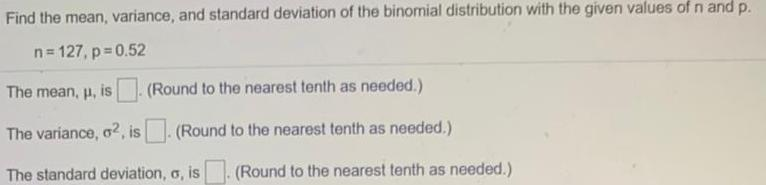Question:

# Find the mean, variance, and standard deviation of theFind the mean, variance, and standard deviation of the binomial distribution with the given values of n and p. n=127, p=0.52